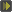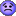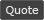WoWInterface - View Single Post - McRun - Official thread
07-15-10, 07:30 PM   #44
Slakah
A Molten Giant
Join Date: Aug 2007
Posts: 863
 Originally Posted by ffcloud2000the Z keybind seems to be the only key that wants to work properly.. all the other keybinds after seem to start back at actionid1 like i'd have to assign each id before the bindfor example i changed the first 4 keybinds to Code: ```bars={ {B=24,W=12,V=-500,H=0, K={='Z',='X',='SHIFT-Q',='SHIFT-E', 'SHIFT-W','SHIFT-A','SHIFT-S','SHIFT-D', 'F1','F2','F3','F4'} }}```
Couldn't you instead just create 2 bars and only assign the keybindings to the second one.
Also instead of using nil, because the settings are kept out of global scope you can just use a nil variable i.e.
lua Code:
`bars={ {B=24,W=12,V=-500,H=0,  K={nil,nil,nil,nil,nil,nil,     nil,nil,nil,nil,nil,nil,     'Z','X','SHIFT-Q','SHIFT-E',     'SHIFT-W','SHIFT-A','SHIFT-S','SHIFT-D',     'F1','F2','F3','F4',}, },}`

would become
lua Code:
`bars={ {B=24,W=12,V=-500,H=0,  K={n,n,n,n,n,n,     n,n,n,n,n,n,     'Z','X','SHIFT-Q','SHIFT-E',     'SHIFT-W','SHIFT-A','SHIFT-S','SHIFT-D',     'F1','F2','F3','F4',}, },}`
ofcourse n could be any variable (r,x,y,z etc.)